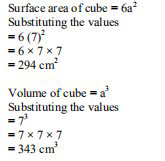# ML Aggarwal Solutions Class 9 Mathematics Solutions for Mensuration Exercise 16.4 in Chapter 16 - Mensuration

Question 1 Mensuration Exercise 16.4

Find the surface area and volume of a cube whose one edge is 7 cm.

It is given that

One edge of cube a = 7 cm

We know thatVideo transcript
"hello and welcome my dear students i am rishabh the match uteron leader and i'm here the new question it says find the surface area and the volume of a cube whose one edge is seven centimeter so without wasting much time let's have a look at the solution it is given that edge of a cube is equal to 7 centimeter and we are supposed to find out the surface area and the volume we know that the surface area is equals to surface area of q is equal to 6 into side square and we are being given the age of a cube as 7 so we'll substitute the value 6 into 7 square is nothing but 294 centimeter square and now we are supposed to find out the volume so volume of a cube is equal to side q and we have been given the side as 7 so we'll substitute the value so we get 7 cube which is nothing but equal to 7 into 7 into 7 which is equals to 343 centimeter cube so i hope my students got this question well do subscribe our channel for the regular updates also you can share your doubts in the comment section see you next time bye"
Related Questions

Lido

Courses

Teachers

Book a Demo with us

Syllabus

Maths
CBSE
Maths
ICSE
Science
CBSE

Science
ICSE
English
CBSE
English
ICSE
Coding

Terms & Policies

Selina Question Bank

Maths
Physics
Biology

Allied Question Bank

Chemistry
Connect with us on social media!